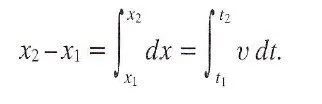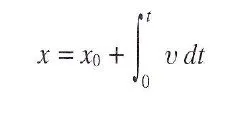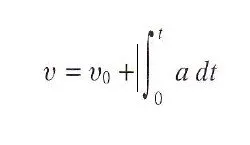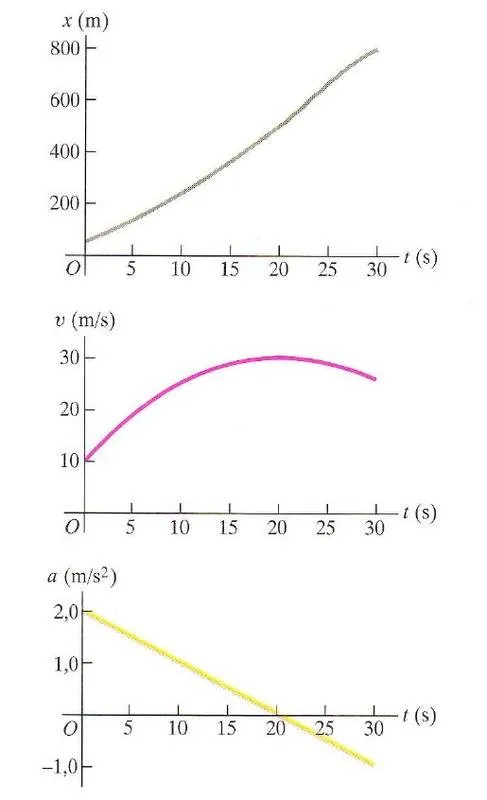# Physics - Classical Mechanics - Rectlinear motion with variable acceleration and velocity relativityHello it's a me again drifter1! Today we continue with Physics and more specifically Classical Mechanics, getting into rectlinear motion with variable acceleration and velocity relativity. We already talked about constant acceleration in the previous post that you can find here and you should check out this and the other posts first before getting into this post. So, without further do, let's get started!

## Integration of velocity and acceleration

We talked about rectlinear motion where the acceleration is constant. With constant acceleration the change in velocity in each second equals the average velocity: Δx / Δt = u avg. What if the acceleration changes? Well, then the velocity change also changes and so the distance we travel in every second.

If we think about the change of acceleration in the same way as we thought of area in integration we can end up with the following:where the change in position (x2 - x1) equals the integral of "small" changes in position dx that are being added together as small rectangle-areas to give us the whole change. Changing the variable with time instead of position we can integrate the velocity with time to get the position.

Doing the same with velocity we can end up with an integral that contains acceleration.You can see that the change in velocity equals the integral of small changes in velocity and afterwards also equals to the integral of acceleration with time.

If we know some starting position or velocity and cause the time is mostly being count from 0 to some value of time t, we can change the two integrals with the following:Integral to find position from velocityIntegral to find velocity from acceleration

### Example

Suppose we know that the acceleration in time is being given by the following function/equation:

a = 2 - 0.1*t, in m/s^2

a) Find the velocity and position functions if at t = 0 sec the object moves with a velocity u0 = 10 m/s and is at the position x0 = 50m

b) Which time t do we have the maximum velocity, what is the value of that velocity and at which position does the object stand at that time?

a)

By doing 2 simple integrations we can find the velocity and then position funciton.

The velocity is:

u = u0 + integral 0->t [2 - 0.1t]dt = 10 + [2t - 0.1/2*t^2 ] 0->1 = 10 + 2t - 0.1/2*t^2 m/s

The position is:

x = x0 + integral 0->t[10 + 2t - 0.1/2*t^2]dt = 50 + [10t + t^2 - 0.1/6*t^3] 0->t

= 50 + 10t + t^2 - 0.1/6*t^3 m

b)

The velocity is maximum at the point where the change in velocity is zero, which means that the acceleration is 0 and so du/dt = a = 0

0 = 2 - 0.1t => t = 2/0.1 = 20s

If we now put the value of time at the velocity and position equations we can find the maximum velocity and the position the object stands at that time.

u max = 10 + 2*20 - 0.1/2*20^2 = 30 m/s

x at max = 50 + 10*20 + 20^2 - 0.1/6*20^3 = 517m

In function diagrams this looks like this:## Relativity of velocity

The final and last thing about rectlinear motion, before getting into more complicated exercises next time, is that velocity is relative.

Suppose that P is an object that moves correspondingly to B, that moves corresponding to object A. Let's say that the relative velocity of P to B is u P/B , the relative velocity of P to A is u P/A and the relative velocity of B to A is u B/A. Can we get an equation that connects all those velocities together?

By doing some math we end up with the following:

u P/A = u P/B + u B/A that is an very useful equation!

Any of those 3 objects can also be stationary!

### Example

Suppose two objects move to each other. Let's say those are Y and T correspondingly. Y is moving up with an velocity of 88km/h and T is moving down with an velocity of 104 km/h. Which is the relative velocity of T to Y?

If we also symbolize E as an stanionary object and represents the "axis" we can say the following:

u Y/E = + 88 km/h and u T/E = - 104 km/h

By using the equation of previously we can now find the relative velocity of Y to T.

u T/E = u T/Y + u Y/E => u T/Y = u T/E - u Y/E = -104 - 88 = -192 km/h which means that the object Y moves down 192 km/h corresponding to object T!

The relative velocity of Y to T is exactly the inverse and equals:

u Y/T = - u T/Y = -(-192 km/h) = + 192 km/h

which means that object T moves 192 km/h up corresponding to object Y!

And this is actually it for today and I hope you enjoyed it!

Next time we will get into some more complicated exercises and examples that will help you understand rectilinear motion even better and after that we will get into plane and circular motion!

Bye!

H2
H3
H4
3 columns
2 columns
1 column
1 Comment